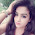## Sunday, January 14, 2018

### Curve Fitting: Fitting to the Curve y = a*e^(-b*x^2)

Curve Fitting:  Fitting to the Curve y = a*e^(-b*x^2)

Introduction

This blog is to fit data to the equation y = a*e^(-b*x^2).  A famous example is the equation:

y = 2/(π) * e^(-x^2)

which the indefinite integral of 0 to real number t is the basis of the error function.

Turning y = a*e^(-b*x^2) into a Linear Form

y = a*e^(-b*x^2)

ln y = ln (a*e^(-b*x^2))

ln y = ln a + (-b)*x^2

Y’ = A’ + B’ * x^2

Where:  Y’ = ln y,  A’ = ln a, B’ = -b

Results:  e^A, B

Inputs:  square each of the data points of X, take the natural logarithm for Y.

Example

I use the HP Prime for this example.

Fit the data below to the curve y = a*e^(-b*x^2).

 x y -0.75 0.642931 -0.35 0.998284 0 1.128379 0.35 0.998284 0.62 0.768267 0.94 0.466951

Adjust data:

 x C1 = x^2 y C2 = ln y (to 6 decimal places) -0.75 0.5625 0.642931 -0.441718 -0.35 0.1225 0.998284 -0.001717 0 0 1.128379 0.120782 0.35 0.1225 0.998284 -0.001717 0.62 0.3844 0.768267 -0.263618 0.94 0.8836 0.466951 -0.761531

Running the linear_regression(C1^2, LN(C2)) returns the matrix [B’, A’]:

[-0.998758, 0.120567]

The results must be adjusted: e^(.120567) = 1.128136

Final adjusted curve:  y ≈ 1.128136 * e^(-0.998758*x^2)

Using the Exponential Regression Model

We can use exponential regression function as an alternative to the linear regression.

For the input, square all of the x data.

 x C1 = x^2 C2 = y -0.75 0.5625 0.642931 -0.35 0.1225 0.998284 0 0 1.128379 0.35 0.1225 0.998284 0.62 0.3844 0.768267 0.94 0.8836 0.466951

The function exponential_regression from the HP Prime that fits data to the model y = B * A^x.

To fit this model asked, y = a*e^(-b*x^2), we will need to take the natural logarithm of B.

Hence:  exponential_regression(C1^2, C2) returns

[0.368337, 1.128136]

With ln(0.368337) ≈ -0.998757, the required equation is

y = 1.128136*e^(-0.998757*x^2)

Summary

To fit data to the curve y = a*e^(-b*x^2):

 Linear Regression, Y’ = A’ + B’*X’ Exponential Regression, Y’ = B’*A’^X’ Input:  X’ = x^2, Y’ = ln y Output:  a = e^A’, b = B’ Input: X’ = x^2, Y’ = y Output: a = A’, b = ln(B’)

Eddie

This blog is property of Edward Shore, 2018.

#### 2 comments:

1.The Correlation Calculator displays correlations for major, exotic and cross currency pairs. It is a useful analytical tool that helps in making a sound trading decision.

2.### HP 41/DM41L and TI-60X: Exponentiation of Large Numbers

HP 41/DM41L  and TI-60X:  Exponentiation of Large Numbers But Why a Program when we have Button? This is true.  What this program does ...